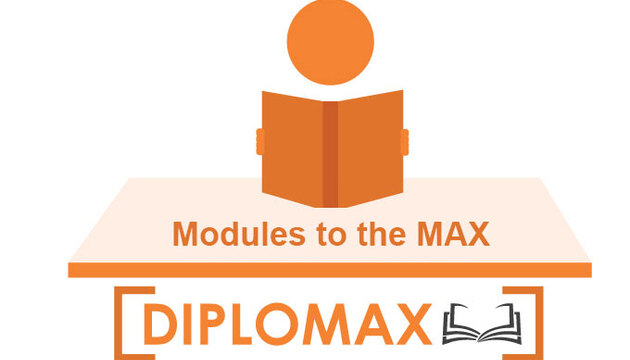Math 30-1 and Modules to the Max

# Math 30-1 - Modules to the MAX

Course by: Diplomax

SKU: 6390#### Description

Available immediately – other subjects under development

• IMMEDIATE, UNLIMITED USE
• Complete curriculum coverage through 25 individual video modules on the topics of:
• Arc Length Questions
• Binomial Theorem
• Combinations with Restrictions
• Converting Forms
• Exponential and Logarithmic Graphs
• Factor Theorem
• Finding the Asympotes and Point Discontinuity
• Function Composition
• Function Operations
• Graphing a Polynomial
• Multiple Transformations
• Permutations with Restrictions
• Reflections and Inverse Restrictions on the Domain
• Remainder Theorem and Polynomial Division
• Solving Combinatorial Equations
• Solving Exponential Equations with Like Bases
• Solving Exponential Equations with Unrelated Bases
• Solving Linear and Quadratic Trig with Varied Domains
• Solving Logarithmic Equations
• Solving Trigonometric Equations with Identities
• The Fundamental Counting Principle
• Transformations Forward and Backward
• Word Problems in Trigonometry
• Word Problems with Exponents and Logs

Each module contains

• 15-20 minutes of instructor video review of each of the 25 topics
• Several step-by-step examples, complete with “worked” solutions
• PDF copies of the practice questions can be saved electronically or printed